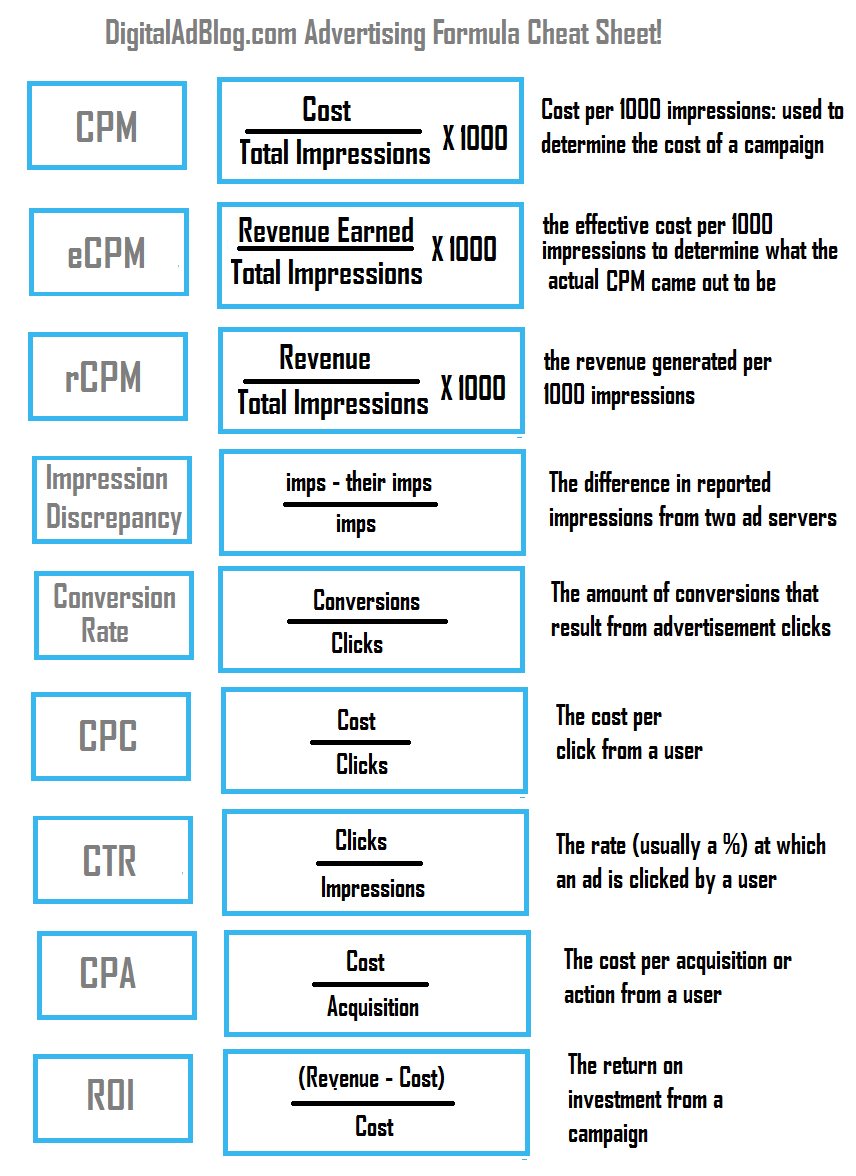# Digital Advertising Formulas Cheat Sheet

Author: | Posted in Formulas No comments

# Make digital advertising simple with this easy to use cheat sheet!

There are many formulas in digital advertising that you should remember to be a successful internet entrepreneur. Here is a handy digital advertising formulas cheat sheet for the basic calculations you will use every day. Share it, print it, tape it to your desk! You will save tons of time and aggravation by having these formulas handy.## Digital Advertising Formulas Cheat Sheet Definitions

CPM is calculated by taking the cost divided by the total impressions times 1000 and is used to determine the cost of a campaign

eCPM is calculated by taking the revenue earned divided by total impressions times 1000 and is what the actual CPM came out to be

rCPM is calculated by taking the revenue divided by total impressions times 1000 and the revenue generated per 1000 impressions

Impressions Discrepancy is calculated by taking your impression count minus the 3rd party impression count divided by your impression count

Conversion Rate is calculated by diving the conversions over the clicks of a campaign and is the amount of conversions that result from a click

CPC which stands for cost per click is calculated by taking the cost divided by the # of clicks

CTR is the click through rate of a campaign and is calculated by taking the clicks divided by the impressions

CPA – Stands for cost per action or acquisition and is cost divided by the number of actions

ROI – Stands for return on investment is calculated by taking the revenue minus the cost divided by the cost.